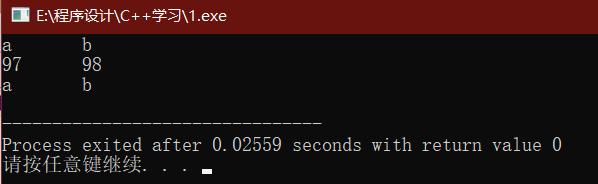•闪存
•博客
• 发言小组
• 投递新闻
• 提问博问
• 添加收藏
•文库

# 局部变量是否释放难道跟数据类型有关吗？

1悬赏园豆：5 [已解决问题] 解决于 2020-06-10 15:51``````char* mul_char2(char *A, char *B)
{
char M;
char *p = M;
M = A * B;
M = A * B;
return p;
}
``````

``````char* mul_char1(char *A, char *B)
{
char M;
M = A * B;
M = A * B;
return M;
}

int* mul_int(int *A, int *B)
{
int M;
int *p = M;
M = A * B;
M = A * B;
return p;
}
``````

1. 以 mul_char2 中为什么加上 char *p = M`就没了警告，而且返回结果正确？它不应该也一样被释放吗？
2. 既然 int 结果不对，那为什么 char2 结果就对呢？局部变量是否释放难道跟变量的类型也有关吗？

``````#include <iostream>
using namespace std;

char* mul_char1(char *A, char *B)
{
char M;
M = A * B;
M = A * B;
return M;
}

char* mul_char2(char *A, char *B)
{
char M;
char *p = M;
M = A * B;
M = A * B;
return p;
}

int* mul_int(int *A, int *B)
{
int M;
int *p = M;
M = A * B;
M = A * B;
return p;
}

int main(int argc, char const *argv[])
{
int A1[] = {97, 98};
int B1[] = {1, 1};
char A2[] = {97, 98};
char B2[] = {1, 1};
char *p = mul_char2(A2, B2);
cout << p << '\t' << p << endl;
int *q = mul_int(A1, B1);
cout << q << '\t' << q << endl;
p = mul_char1(A2, B2);
cout << p << '\t' << p << endl;
return 0;
}
``````
``````> Executing task: D:\mingw-w64\x86_64-8.1.0-win32-seh-rt_v6-rev0\mingw64\bin\g++.exe -g c:\Users\dongx\Desktop\test\C++\main.cpp -o c:\Users\dongx\Desktop\test\C++\main.exe <

c:\Users\dongx\Desktop\test\C++\main.cpp: In function 'char* mul_char1(char*, char*)':
char M;
^

Terminal will be reused by tasks, press any key to close it.

a       b
97      0
The terminal process terminated with exit code: 1

Terminal will be reused by tasks, press any key to close it.
``````

1
1. 你返回的是一个地址
2. 这个地址是线程中栈里面的一个地址
3. 虽然在函数结束时局部变量释放了，可以这个地址是真实存在的，而且这个地址对于的值也还在。
4. 如果你在函数结束后调用了其他函数，然后执行一段代码之后再访问这个指针的值你就会发现，值很可能就不正确了。因为栈被反复使用了，里面的值早就变了。

jiajia_ | 菜鸟二级 |园豆：234 | 2020-06-08 19:06

0Conan-jine | 园豆：1272 (小虾三级) | 2020-06-05 18:47

您需要登录以后才能回答，未注册用户请先注册